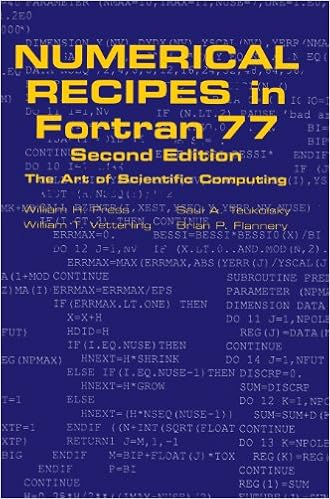# Fortran Wiki Numerical Recipes in Fortran 77By William H. Press, Brian P. Flannery, Saul A. Teukolsky, William T. Vetterling (1992)
Cambridge University Press## Description

This is the revised and expanded second edition of the hugely popular Numerical Recipes: the Art of Scientific Computing. The product of a unique collaboration among four leading scientists in academic research and industry, Numerical Recipes is a complete text and reference book on scientific computing. In a self-contained manner, it proceeds from mathematical and theoretical considerations to actual, practical computer routines. With over 100 new routines, bringing the total to well over 300, plus upgraded versions of many of the original routines, this second edition, first published in 1993, is the most practical, comprehensive handbook of scientific computing available today. The book retains the informal, easy-to-read style that made the first edition so popular, even while introducing some more advanced topics. It is an ideal textbook for scientists and engineers, and an indispensable reference for anyone who works in scientific computing. The second edition is available in FORTRAN, the quintessential language for numerical calculations, and in the increasingly popular C language.

## Contents

1. Preliminaries
2. Solution of linear algebraic equations
3. Interpolation and extrapolation
4. Integration of functions
5. Evaluation of functions
6. Special functions
7. Random numbers
8. Sorting
9. Root finding and nonlinear sets of equations
10. Minimization or maximization of functions
11. Eigensystems
12. Fast Fourier transform
13. Fourier and spectral applications
14. Statistical description of data
15. Modeling of data
16. Integration of ordinary differential equations
17. Two point boundary value problems
18. Integral equations and inverse theory
19. Partial differential equations
20. Less-numerical algorithms

category: books